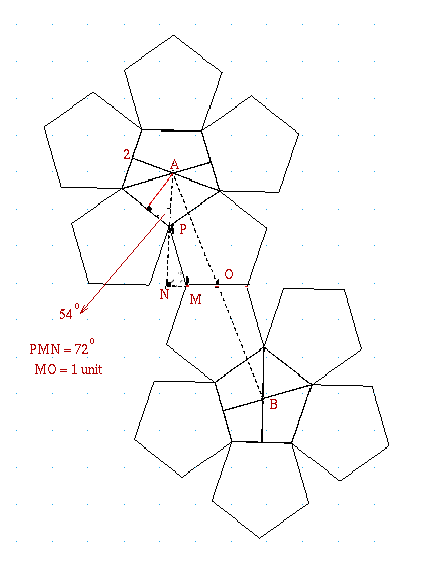#### You may also like### Degree Ceremony

What does Pythagoras' Theorem tell you about these angles: 90°, (45+x)° and (45-x)° in a triangle?### Logosquares

Ten squares form regular rings either with adjacent or opposite vertices touching. Calculate the inner and outer radii of the rings that surround the squares.### Ball Bearings

If a is the radius of the axle, b the radius of each ball-bearing, and c the radius of the hub, why does the number of ball bearings n determine the ratio c/a? Find a formula for c/a in terms of n.

# The Dodecahedron

##### Age 16 to 18 Challenge Level:

There are two interpretations of this problem, the shortest route between opposite faces of the dodecahedron going across the outside of the solid and the shortest route boring a hole through the middle.

While few tackled this challenging problem, Luke, of Madras College found the shortest distance through the centre. In his solution he used the centres of three spheres: the circumsphere that passes through all the vertices of the dodecahedron, the midsphere touching the midpoints of the edges, and the insphere which touches the opposite faces of the dodecahedron at their centres. Luke's answer is 2.23 approximately. Before you look at Luke's solution here you might like to try to try it for yourself.

On the surface this must be the distance between A and B on the net of the solid.Let the lengths of the edges be 2 units.
AB = 2 x AO
AO = ON + AN
and AN = AP + PN.

Working to 4 decimal places,
AP = 1/cos 54° = 1.7015
PN = 2 sin72° = 1.9021
NM = 2 cos72° = 0.6180

AN = 1/cos54° + 2sin72° = 3.6034
NO = 2cos72° + 1 = 1.6180

AO² = (1.6180)² + (3.6034)²
AO = 3.9499 AB = 7.8999